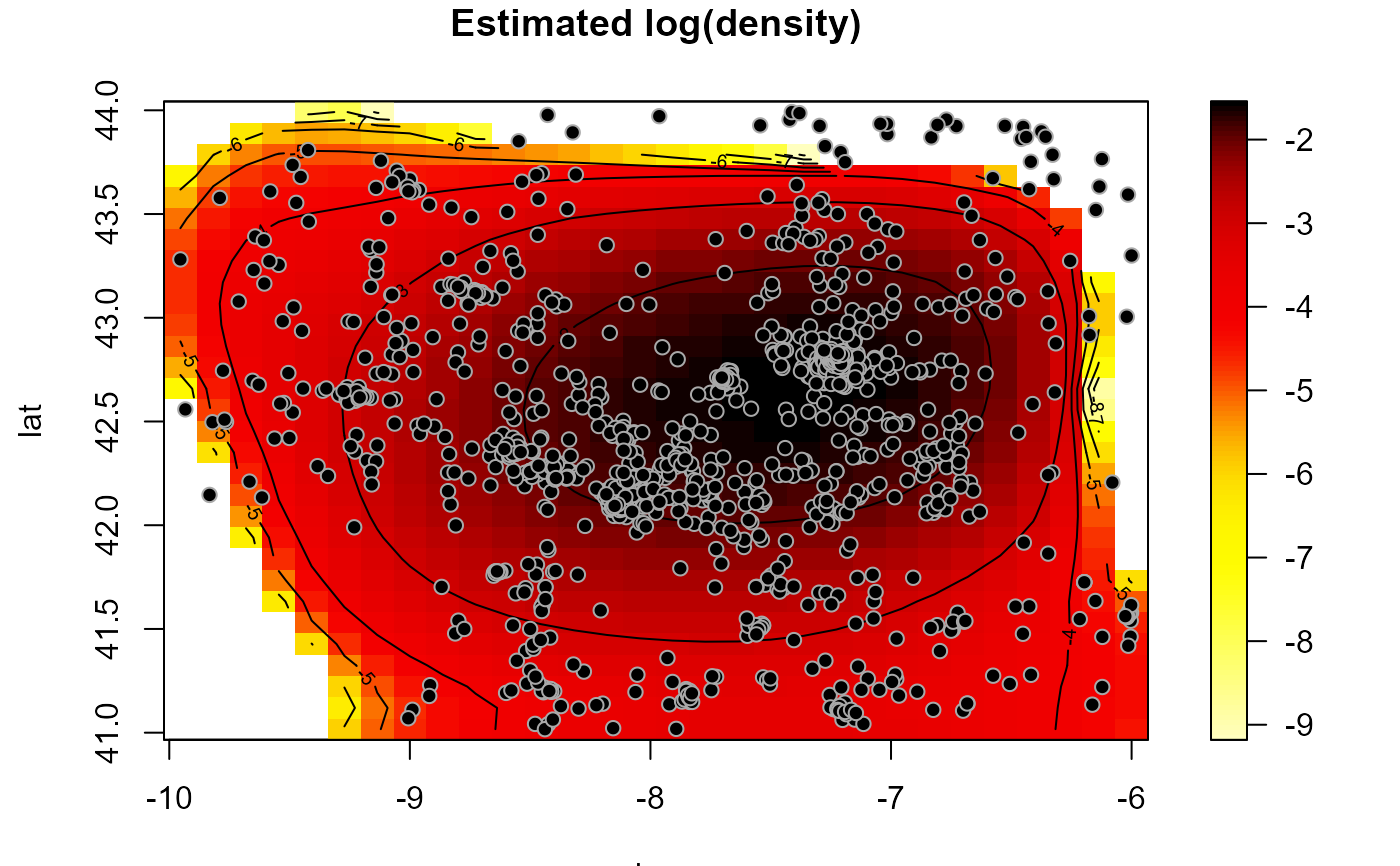Estimates a multidimensional probability density function (and its first derivatives) using local polynomial kernel smoothing of linearly binned data.

np.den(x, ...)

# S3 method for default
np.den(
x,
nbin = NULL,
h = NULL,
degree = 1 + as.numeric(drv),
drv = FALSE,
ncv = 0,
...
)

# S3 method for bin.den
np.den(x, h = NULL, degree = 1 + as.numeric(drv), drv = FALSE, ncv = 0, ...)

# S3 method for bin.data
np.den(x, h = NULL, degree = 1 + as.numeric(drv), drv = FALSE, ncv = 0, ...)

# S3 method for svar.bin
np.den(x, h = NULL, degree = 1 + as.numeric(drv), drv = FALSE, ncv = 0, ...)

## Arguments

x a (data) object used to select a method. further arguments passed to or from other methods. vector with the number of bins on each dimension. (full) bandwidth matrix (controls the degree of smoothing; only the upper triangular part of h is used). degree of the local polynomial used. Defaults to 1 (local linear estimation). logical; if TRUE, the matrix of estimated first derivatives is returned. integer; determines the number of cells leaved out in each dimension. Defaults to 0 (the full data is used) and it is not normally changed by the user in this setting. See "Details" below.

## Value

Returns an S3 object of class np.den (locpol den + bin den + grid par.). A bin.den object with the additional (some optional) 3 components:

est

vector or array (dimension nbin) with the local polynomial density estimates.

locpol

a list with 6 components:

• degree degree of the polinomial.

• h bandwidth matrix.

• rm residual mean (of the escaled bin counts).

• rss sum of squared residuals (of the escaled bin counts).

• ncv number of cells ignored (in each dimension).

deriv

(if requested) matrix of first derivatives.

## Details

Standard generic function with a default method (interface to the fortran routine lp_data_grid), in which argument x is a vector or matrix of covariates (e.g. spatial coordinates). In this case, the data are binned (calls bin.den) and the local fitting procedure is applied to the scaled bin counts (calls np.den.bin.den).

If parameter nbim is not specified is set to rep(25, ncol(x)).

A multiplicative triweight kernel is used to compute the weights.

If ncv > 1, estimates are computed by leaving out cells with indexes within the intervals $$[x_i - ncv + 1, x_i + ncv - 1]$$, at each dimension i, where $$x$$ denotes the index of the estimation position.

Wand, M.P. and Jones, M.C. (1995) Kernel Smoothing. Chapman and Hall, London.

bin.den, binning, data.grid.
bin <- binning(earthquakes[, c("lon", "lat")], earthquakes$mag, nbin = c(30,30)) hden <- h.cv(as.bin.den(bin)) den <- np.den(bin, h = hden$h)